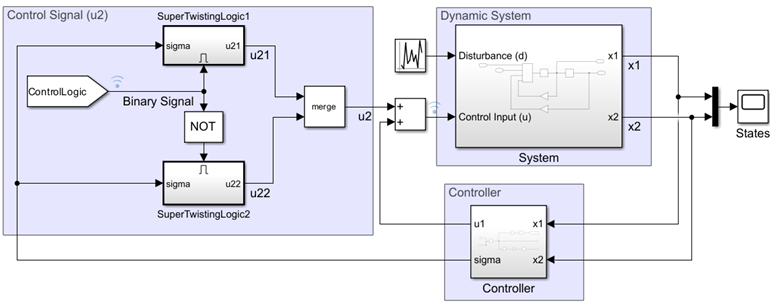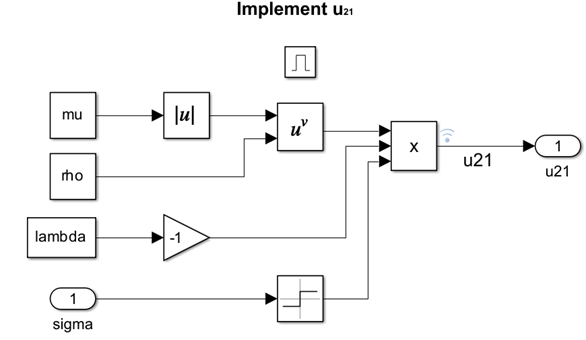# Enabled Subsystems

This example shows what happens when a sine wave is fed into an enabled subsystem. After running the simulation, the scope shows three plots.

The first of these plots shows the original sine wave and the absolute value of the original sine wave offset by two. The absolute value of the sine wave is shown only when the subsystem is enabled. This occurs between 0 and 2.5 seconds, and between 5 and 7.5 seconds. When the subsystem is not enabled, the output of the subsystem is reset to 0. The value of 0 is determined by the initial condition of the outport. You can see when the subsystem is enabled by looking at the second plot. A value of 1 indicates that it is enabled.

The second plot shows the original value of the enable signal. When the signal is towards the top of the plot, the subsystem related to the first plot is enabled. When the signal is towards the bottom of the plot, the subsystem related to the third plot is enabled. The difference in when the subsystems are enabled is caused by the logical NOT block.

The third plot shows the original sine wave and the saturation limited sine wave offset by two. The saturated value of the sine wave is shown only when the subsystem is enabled. This occurs between 2.5 and 5 seconds, and between 7.5 and 10 seconds.